[toc]

## slice 的 length 和 capacity

``````s := make([]int, 3, 6)
``````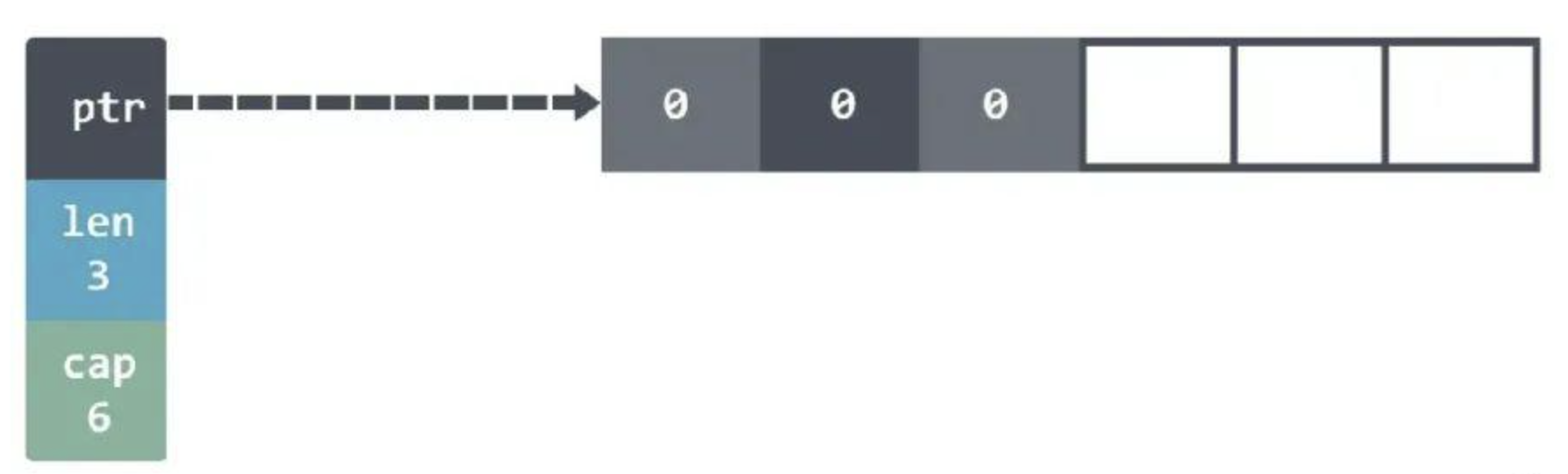slice 的底层实际上指向了一个数组。当然，由于我们的 length 是 3，所以这样设置 s = 0 会 panic 的。需要使用 append 才能添加新元素。

``````panic: runtime error: index out of range  with length 3
``````

``````s1 := make([]int, 3, 6)
s2 := s1[1:3]
``````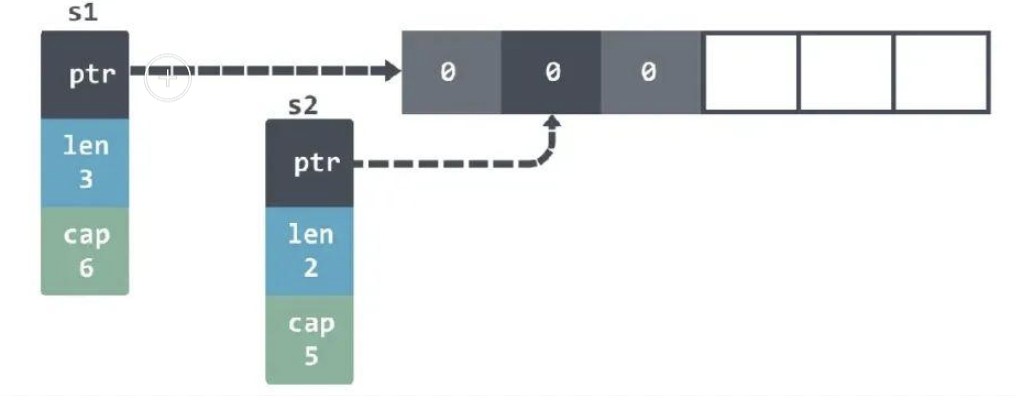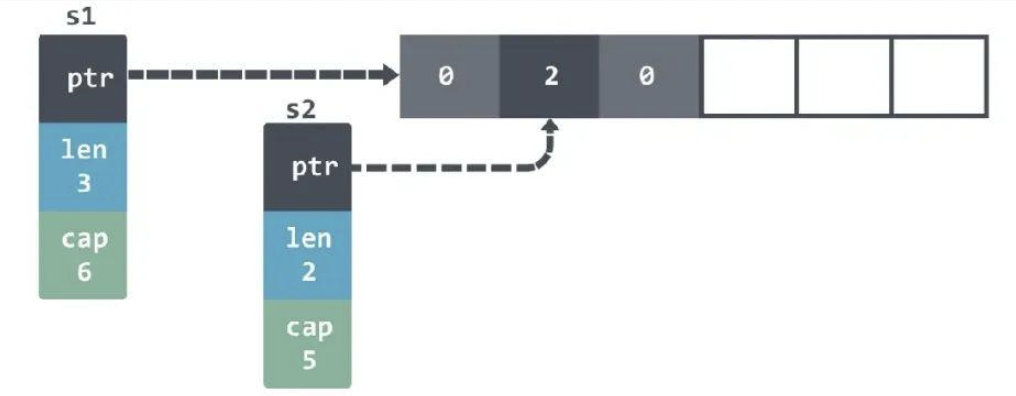``````s2 = append(s2, 3)

fmt.Println(s1) // [0 2 0]
fmt.Println(s2) // [2 0 3]
``````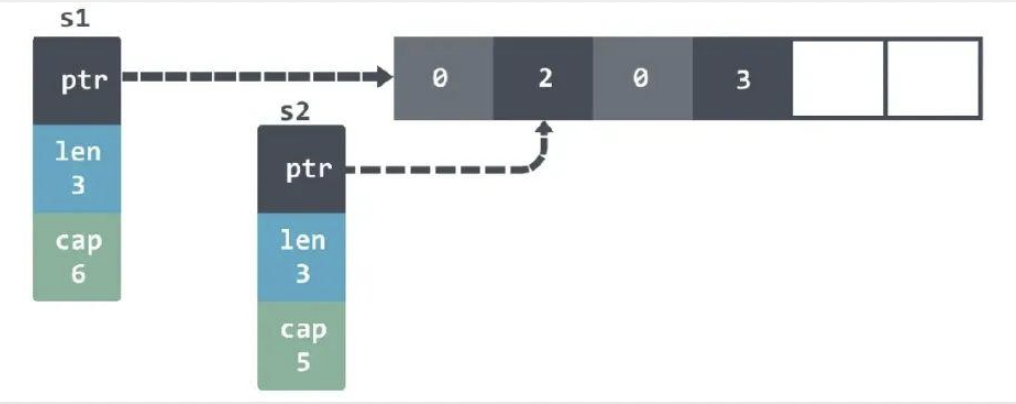s1 的 len 并没有被改变，所以看到的还是3元素。

``````s1 = append(s1, 4)
fmt.Println(s1) // [0 2 0 4]
fmt.Println(s2) // [2 0 4]
``````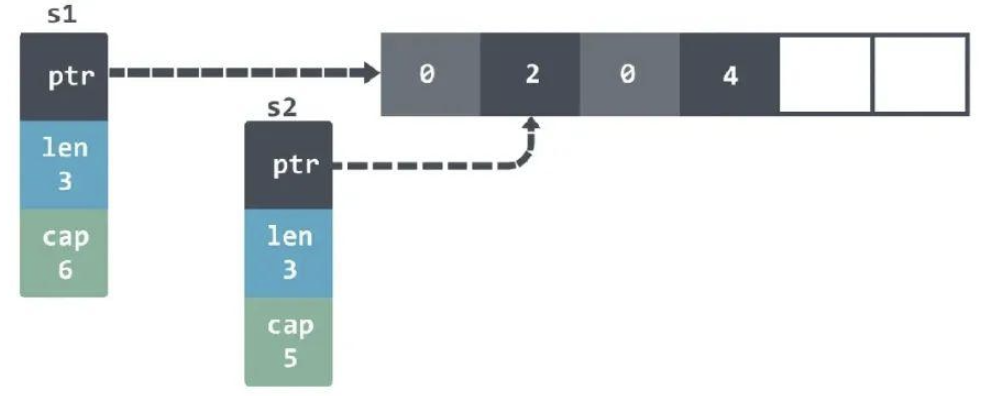``````s2 = append(s2, 5, 6, 7)
fmt.Println(s1) //[0 2 0 4]
fmt.Println(s2) //[2 0 4 5 6 7]
``````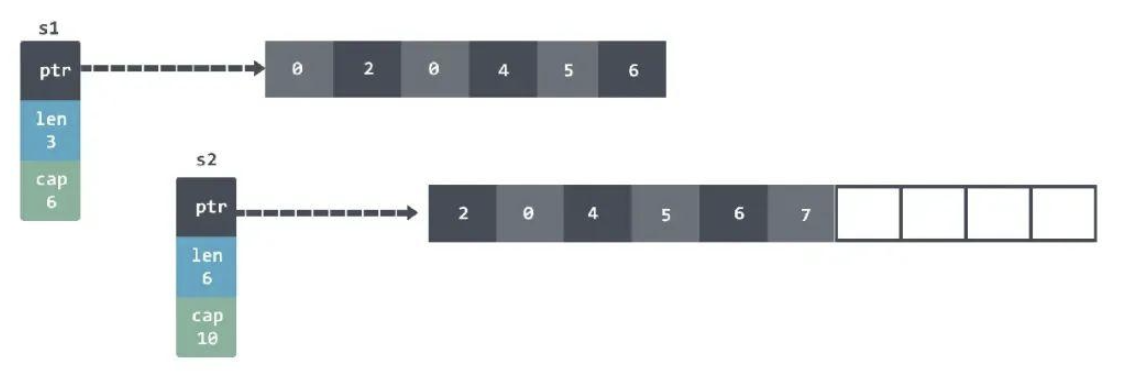``````s1 := []int{1, 2, 3}
s2 := s1[1:2]
s3 := append(s2, 10)
``````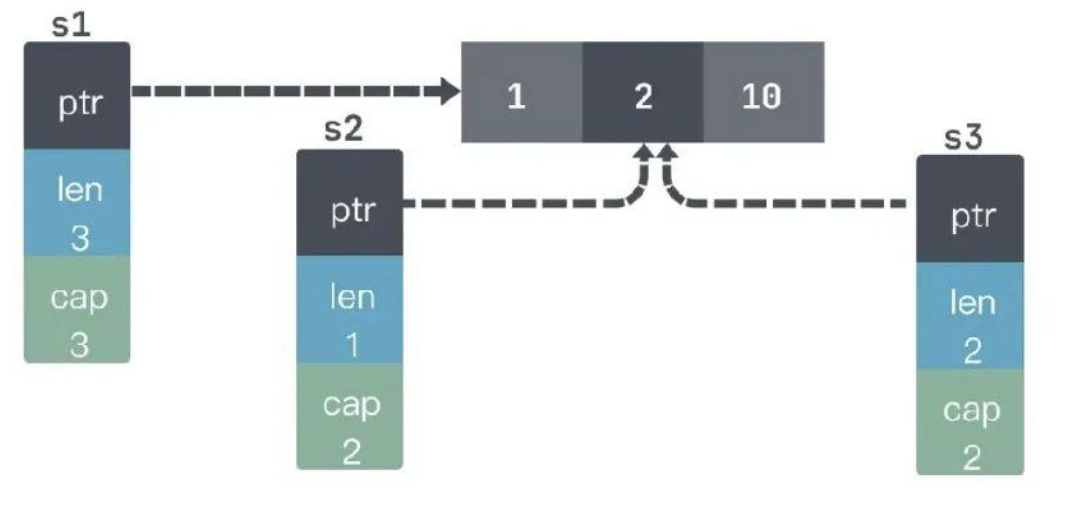``````s1=[1 2 10], s2=, s3=[2 10]
``````

## slice 初始化

slice 的初始化有很多种方式：

``````func main() {
var s []string
log(1, s)

s = []string(nil)
log(2, s)

s = []string{}
log(3, s)

s = make([]string, 0)
log(4, s)
}

func log(i int, s []string) {
fmt.Printf("%d: empty=%t\tnil=%t\n", i, len(s) == 0, s == nil)
}
``````

``````1: empty=true   nil=true
2: empty=true   nil=true
3: empty=true   nil=false
4: empty=true   nil=false
``````

``````func f() []string {
var s []string
if foo() {
s = append(s, "foo")
}
if bar() {
s = append(s, "bar")
}
return s
}
``````

``````s := []string{"foo", "bar", "baz"}
``````

``````src := []int{0, 1, 2}
dst := append([]int(nil), src...)
``````

``````BenchmarkConvert_EmptySlice-4 22 49739882 ns/op
BenchmarkConvert_GivenCapacity-4 86 13438544 ns/op
BenchmarkConvert_GivenLength-4 91 12800411 ns/op
``````

## copy slice

``````src := []int{0, 1, 2}
var dst []int
copy(dst, src)
fmt.Println(dst) // []
``````

``````src := []int{0, 1, 2}
dst := make([]int, len(src))
copy(dst, src)
fmt.Println(dst) //[0 1 2]
``````

``````src := []int{0, 1, 2}
dst := append([]int(nil), src...)
``````

## slice capacity内存释放问题

``````type Foo struct {
v []byte
}

func keepFirstTwoElementsOnly(foos []Foo) []Foo {
return foos[:2]
}

func main() {
foos := make([]Foo, 1_000)
printAlloc()

for i := 0; i < len(foos); i++ {
foos[i] = Foo{
v: make([]byte, 1024*1024),
}
}
printAlloc()

two := keepFirstTwoElementsOnly(foos)
runtime.GC()
printAlloc()
runtime.KeepAlive(two)
}
``````

``````func printAlloc() {
var m runtime.MemStats
fmt.Printf("%d KB\n", m.Alloc/1024)
}
``````

``````387 KB
1024315 KB1024319 KB
``````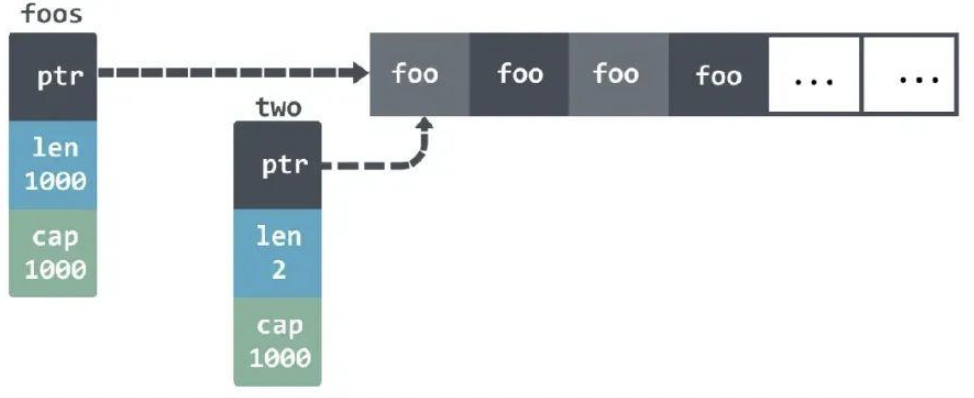``````func keepFirstTwoElementsOnly(foos []Foo) []Foo {
res := make([]Foo, 2)
copy(res, foos)
return res
}
``````

``````func keepFirstTwoElementsOnly(foos []Foo) []Foo {
for i := 2; i < len(foos); i++ {
foos[i].v = nil
}
return foos[:2]
}
``````